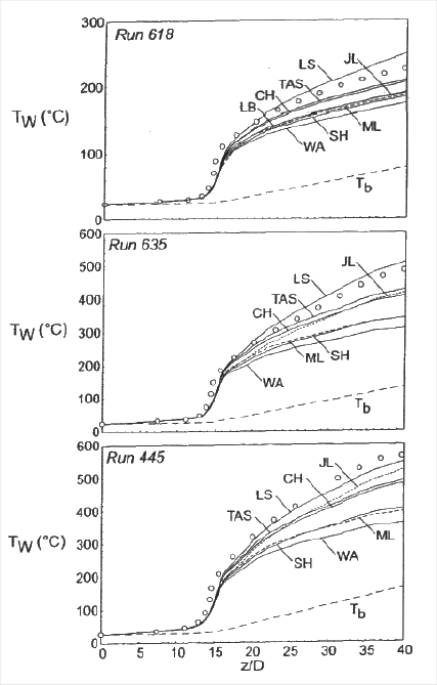# Evaluation

## Comparison of CFD calculations with Experiments

Calculations using the seven low reynolds number (k,ε) models previously described have been made for air and the conditions of runs 445, 635 and 618, yielding a range of flows from essentially turbulent to laminarizing. The inlet pressure and temperature were set to the measured values.

To illustrate the predictions of integral heat transfer, the wall temperature distributions obtained with all the models have been compared for the three runs (Figure 1).

In this figure, the distance z/D is labeled from the tube inlet so the nominal start of heating is at z/D = 14.8 and the near uniform wall heat flux range is in the region 20<z/D<41.

For the lowest heating rate (run 618), the predictions are examined at z/D = 40 where the wall temperature is highest. The wall to bulk temperature difference is under predicted by all models except the LS model. The worst prediction underestimates the temperature difference by over 30% (SH). Forecasts within 20% of the measured values were yielded by Chien and TAS. The LS model did better with a conservative overprediction of about 13%.

Comparisons in the cases of the two higher heating rates (runs 445 and 635) discriminate the models further. The models showing poor agreement at the lower heating rates remain poor and the LS model clearly performed best in each case. The JL model improved relative to the CH and TAS models but the temperature difference at z/D = 40 is underpredicted by over 15%.

Therefore the LS model has been selected for comparisons with further experimental data: profiles of local mean velocity and temperature measured at various axial positions. Figure 2 provides direct comparison between the predicted profiles and the data. In all cases the first predicted velocity profiles near the wall are low relative to the data but show good agreement for y/R>0.1.

At the conditions of turbulent run 618, the predicted velocities show reasonable agreement at x/D = 14 and 25 but are still slightly lower than the data. The velocity predictions of “intermediate” run 635 behave much like those of run 618 with better agreement occurring after the first station, but at x/D = 9 and 14, the predictions are still low and the temperature predictions are high, accordingly. By 14 diameters, the flow appears to be effectively laminarized from the wall to y/R equals about 0.2. By 19 diameters, the velocity agreement is quite good considering the difficult conditions.

For run 445, velocity and temperature predictions look good throughout, despite the fluid property variations being greatest for that case. This result probably occurs because molecular transport increases in importance once the laminarizing process has begun and so the uncertainties in the turbulence modelling become of less significance.

Overall agreement between the LS model predictions and the profile measurements is good though close examination has revealed some detailed discrepancies. For the two higher heating rates, the predictions of temperature differences differed from the data by only 6% which is comparable to the estimated uncertainty of the measurements for these conditions.

``````

Figure 1 (from ref.1): Comparisons of the computed wall temperature distributions and the experimental ones (marked by circles) for seven low-Reynolds k-ε models.

Figure 2 (from ref.1): Comparisons between the predicted profiles by the LS model and the data.